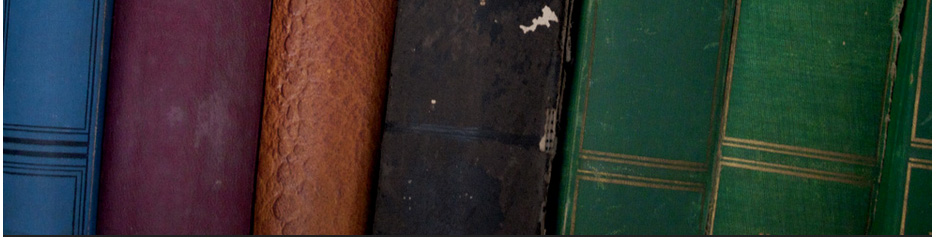## Secondary education# Mathematics KS3

Algebra Geometry
Expressions and formulae Transformations
Equation of straight line &Graphs Symmetry
Patterns and sequences Pythagoras
Solving systems of linear inequalities. Angles and triangles
Equations [solving linear equation] Congruence, similarity, coordinate proofs (SSS, SAS, ASA)
Straight line graph[youtube] Loci and bearing
Simultaneous equations
Statistics and probability

## Coordinate geometry

Powers and roots[square roots, cube roots] Introduction to Euclid's geometry
Irrational and real numbers. Area of 2D and 3D Shape
Exponential Functions ;rules for exponents, Properties of Angles and 2D shape
Exponential and quadratic functions. Constructing traiangles and bisectors
Solving linear systems graphically and algebraically, Circle Theorems
Exercises in KS3 Mathematics

## KS 3-4 Worksheets for All Topics

[Vidoes [Algebra]/ [Vidoes [Geometry]]
Year 9 maths
Converting recurring decimals to fraction Year 7 and 8 Maths for ks3 i

Ermias Home

HAREP The Horn of Africa Research and Knowledge Exchange Platform © 2010-2012